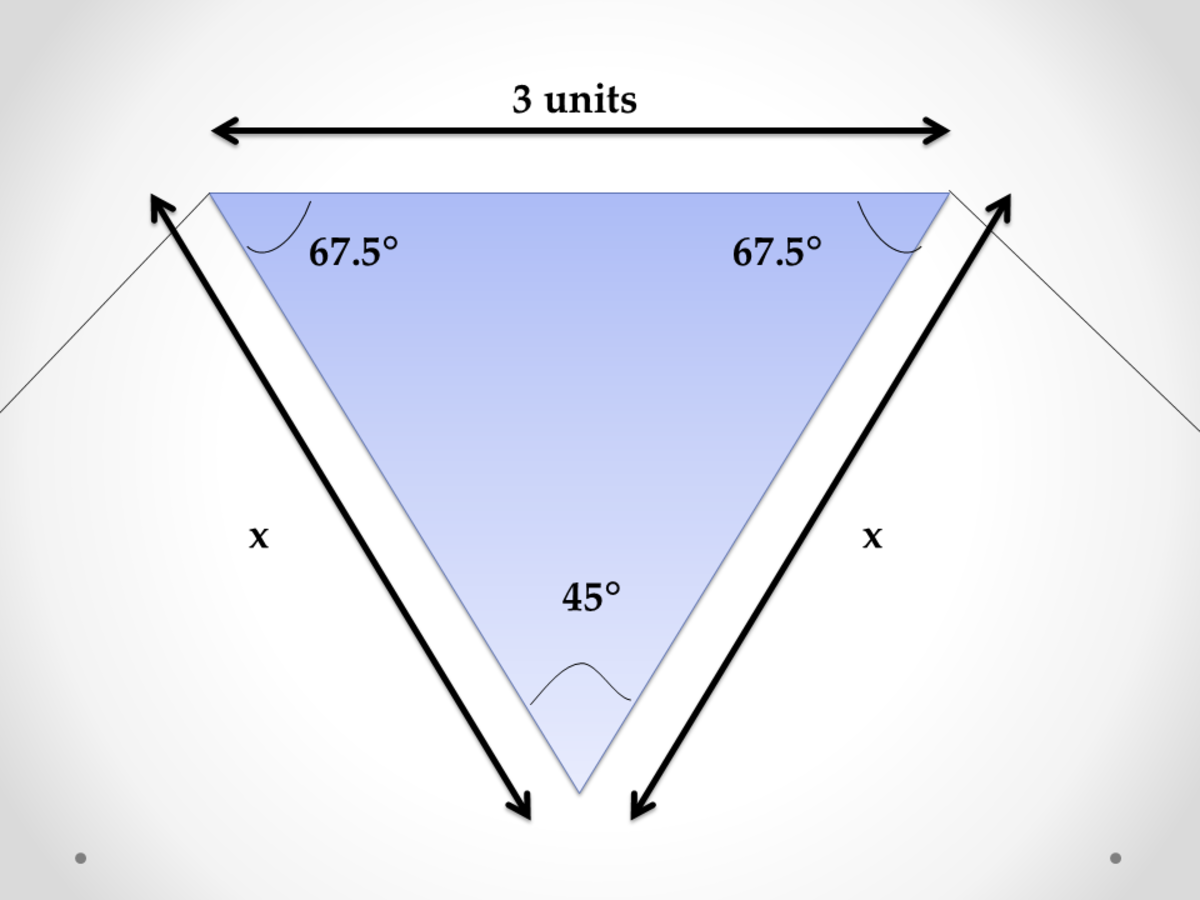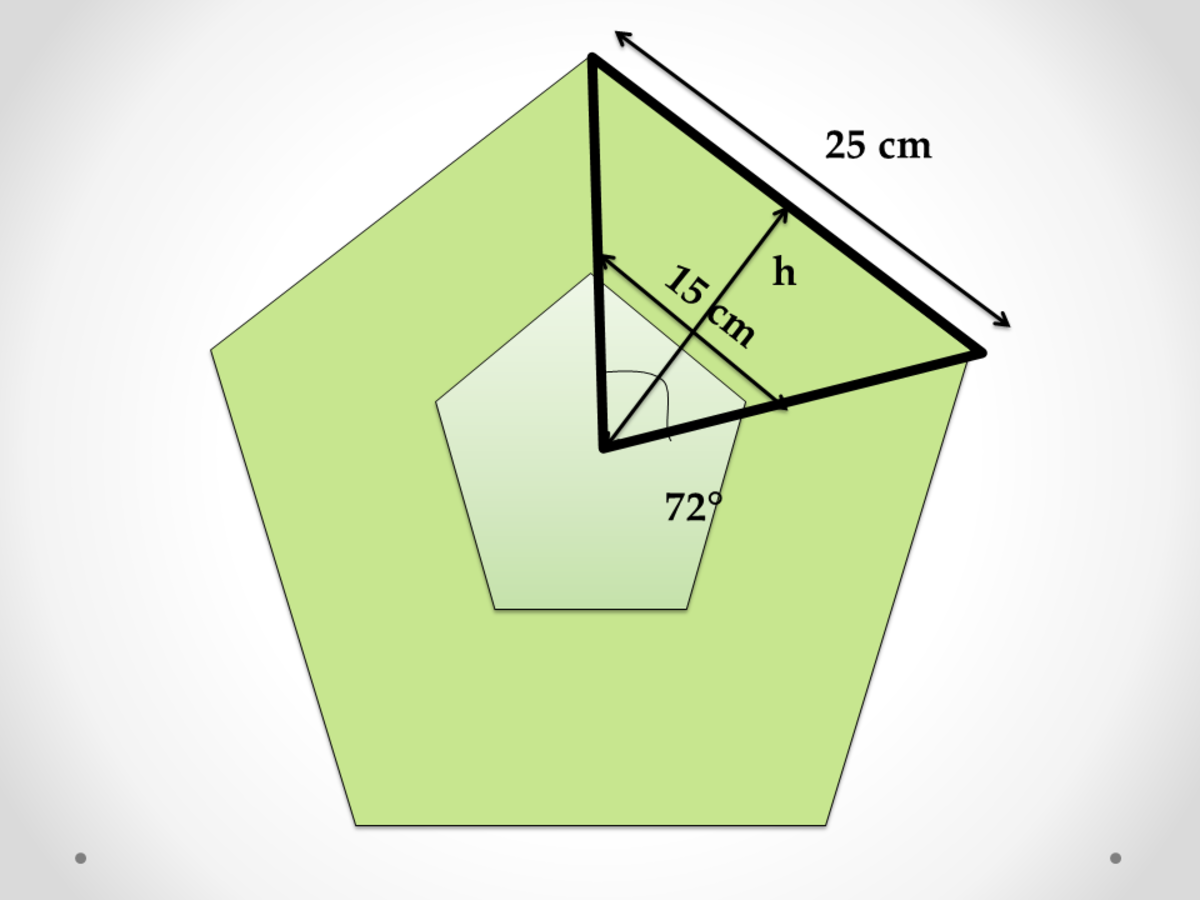# Calculator Techniques for Polygons in Plane Geometry

• Author:
• Updated date:

## What Are Polygons?

Polygons are closed plane surfaces with n number of sides and n number of vertices. There are two types of polygons, a convex and a concave polygon. A convex polygon is a polygon in which no side, when extended, will pass inside the polygon; otherwise, the polygon is called concave.

There are also subcategories for polygons. Equilateral polygons are polygons whose sides are equal, while polygons whose angles are equal are called equiangular polygons. Then, a polygon which is both equilateral and equiangular is called a regular polygon. Polygons have diagonals which are line segments joining two non-adjacent sides of the polygon.

## How to Classify Polygons

Polygons are classified based on the number of sides. Here is a list of different polygons, from the two-sided polygon to the thousand-sided polygon.

Number of SidesName of the PolygonNumber of SidesName of the Polygon

1

No name

16

2

Digon

17

3

Triangle

18

4

19

5

Pentagon

20

Icosagon

6

Hexagon

30

Triacontagon

7

Heptagon

40

Tetracontagon

8

Octagon

50

Pentacontagon

9

Nonagon

60

Hexacontagon

10

Decagon

70

Heptacontagon

11

Undecagon

80

Octacontagon

12

Dodecagon

90

Enneacontagon

13

Tridecagon

100

Hectogon

14

1000

Chilliagon

15

10000

Myriagon

## Calculator Techniques for Polygons

Calculator techniques for problems related to polygons are more on algebra and trigonometry. Memorization of formulas is what is needed. Here are the contents of the article.

• Sum of interior angles of a polygon
• Number of sides of a polygon
• Area of polygons given the length of one side
• Number of diagonals of a polygon
• Number of sides of a polygon given the number of diagonals
• Polygons inscribed in a circle
• Polygons circumscribed a circle
• Five-pointed star inscribed in a circle
• Variation of problems

### Problem 1: Sum of Interior Angles of a Polygon

The sum of the interior angles of a polygon is 1,440. Find the number of sides.

Calculator Technique

a. The formula for solving the sum of the interior angles is:

The polygon has ten sides. Therefore, it is a decagon.

### Problem 2: Number of Sides of Equiangular Polygons

How many sides are in an equiangular polygon if each of its interior angles is 165°?

Calculator Technique

a. Let n be the number of sides. Use the same formula and solve for the number of sides

The equiangular polygon has twenty-four sides. Therefore, it is a Tetra Icosagon.

### Problem 3: Finding the Area of a Polygon Given a Side

One side of a regular octagon is three units. Find the area of the octagon.

Calculator Technique

a. Divide the octagon into eight equal parts. Calculate the interior and exterior angles of the octagon.Finding the area of a polygon given a side in plane geometryJohn Ray Cuevas

b. Use sine law in solving for the remaining sides.

c. Solve for the area of the triangle. Multiply it by the number of sides, which is 8.

The total area of the octagon is 44.34 square units.

### Problem 4: Diagonals of a Polygon Given the Number of Sides

How many diagonals have a dodecagon?

Calculator Technique

a. The formula for solving the diagonals of a polygon is:

The total number of diagonals in a dodecagon is 54.

### Problem 5: Number of Sides of a Polygon Given the Number of Diagonals

The number of diagonals of a polygon is 252. How many sides are there?

Calculator Technique

a. The formula for solving the diagonals of a polygon is:

The total number of sides is 24.

### Problem 6: Number of Sides of a Polygon

How many sides does a polygon have if the sum of its exterior angles equals the sum of its interior angles?

Calculator Technique

a. The formula for solving the sum of the interior angles is:

The total number of sides of a polygon whose sum of its exterior angles equals the sum of its interior angles is 4.

### Problem 7: Area of Big and Small Polygons

A regular pentagon has sides of 25 cm. An inner pentagon with sides of 15 cm is inside and concentric to the larger pentagon. What is the area inside the larger pentagon and outside the smaller pentagon?Area of big and small polygons in plane geometryJohn Ray Cuevas

Calculator Technique

a. Solve for the value of h for both the small and larger triangle formed.

b. Solve for the area of the big and small pentagon.

c. Solve for the area difference between the larger pentagon and smaller pentagon.

The area inside the larger pentagon and outside the smaller pentagon is 688 square centimeters.

### Problem 8: Five-Pointed Star Inscribed in a Circle

Find the sum of the interior angles of the vertices of a five-pointed star inscribed in a circle.

Calculator Technique

a. Solve for the value of the interior angles using the formula:

b. Solve for the sum of interior angles of the vertices.

The sum of the interior angles of a five-pointed star is 180 degrees.

### Problem 9: Area of a Circle Inscribed in a Hexagon

The side of the hexagon is h. Find the area of the biggest circle inscribed in a hexagon.

Calculator Technique

a. Solve for the radius of the circle which is same as the height of the triangle shown.

b. Calculate the area of the circle.

The area of the largest circle inscribed in a hexagon is 2.356h2.

### Problem 10: Equilateral Triangle Inscribed in a Hexagon

Given a variable 'h' as the side of a hexagon, find the area of the equilateral triangle that can be inscribed in a hexagon.